# CBSE Class 10 Maths Chapter 11 Construction Objective Questions

According to the CBSE Syllabus 2023-24, this chapter has been removed from NCERT Class 10 Maths textbook.

CBSE Class 10 Maths Chapter 11 Constructions Objective Questions include topics such as Division of a Line Segment, Constructions of Tangents to a Circle, Line Segment Bisector, and so on. Class 10, Chapter 11 -Constructions is one of the scoring chapters that come under the unit Geometry. Based on the latest modification of the exam pattern, we have compiled here the CBSE Class 10 Maths Chapter 11 – Construction Objective Questions for the students to practise and score well in the board examination.

Find the sub-topics covered in the chapter, based on which the CBSE Class 10 Maths objective questions are formed.

### Sub-topics Covered in Chapter 11

11.1 Constructing Similar Triangles (8 MCQs from the Topic)

11.2 Construction of Tangents to a Circle (3 MCQs from the Topic)

11.3 Drawing Tangents to a Circle (3 MCQs from the Topic)

11.4 Dividing a Line Segment (6 MCQs from the Topic)

Find below the link to access the list of MCQs organised topic-wise from Chapter 11 Constructions of CBSE Class 10 Maths.

## Download CBSE Class 10 Maths Chapter 11 – Construction Objective Questions

### Constructing Similar Triangles

1. The ratio of corresponding sides for the pair of triangles whose construction is given as follows: Triangle ABC of dimensions AB=4cm, BC= 5 cm, and ∠B= 60o. A ray BX is drawn from B, making an acute angle with AB. 5 points B1, B2, B3, B4 and B5 are located on the ray, such that BB1=B1B2=B2B3=B3B4=B4B5. B4 is joined to A, and a line parallel to B4A is drawn through B5 to intersect the extended line AB at A’. Another line is drawn through A’ parallel to AC, intersecting the extended line BC at C’. Find the ratio of the corresponding sides of ΔABC and ΔA′BC′.
1. 1:5
2. 1:4
3. 4:5
4. 4:1

Solution: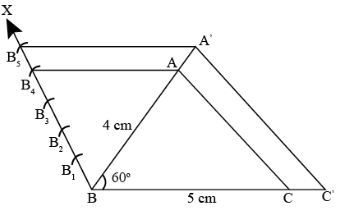According to the construction, ΔBB4A∼ΔBB5A′

And, for similar triangles, the ratio of corresponding sides is AB/A′B.

Hence, the ratio of the corresponding sides is 4:5.

1. If I ask you to construct △PQR ~ △ABC exactly (when we say exactly, we mean the exact relative positions of the triangles) as given in the figure (Assuming I give you the dimensions of △ABC and the scale factor for △PQR), what additional information would you ask for?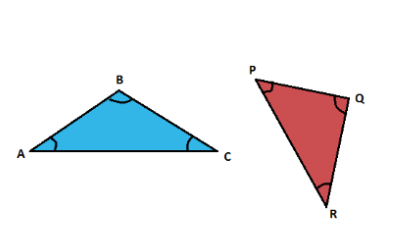1. The information given is sufficient.
2. We cannot construct the triangle because there is no connection between the two triangles.
3. The perpendicular distance between AC and Point P and the angle between AC and PR.
4. Dimensions of PQR.

Answer: (C) The perpendicular distance between AC and Point P and the angle between AC and PR.

Solution: Consider that we need to draw a triangle △PQR ~ △ABC. But △PQR is at an isolated position w.r.t. △ABC. Therefore, we need to know the perpendicular distance between AC and point P and the angle between AC and PR.

Now let us reframe the question with the required information.

Construct a triangle △PQR similar to △ABC, such that point P is at a perpendicular distance of 5 cm from the line AC, PR makes an angle of 60 o and which is 23rd of △ABC. In triangle, △ABC, AB = 6 cm, AC = 7 cm, and ∠ABC = 30o.

Steps of construction:

1. Draw a straight line AC = 7 cm.

2. Measure an angle of 30 o w.r.t AC and draw a straight line.

3. Set the compass at 6 cm in length, and with A as the centre, mark the length on the line, and name the point of intersection as B.

Reason: This is done to draw the line AB, i.e. we are marking the length of 6 cm on the line 30o inclined to AC.

4. Connect the points to BC to complete the triangle.

5. Draw a line perpendicular to AC at A.

Reason: We are drawing this step to identify the line on which point P might lie.

6. For the given length of AP, cut the perpendicular at P.

Reason: We are drawing the arc to identify the exact position of point P on the line perpendicular to AC.

7. Extrapolate line segment AC.

8. Draw a Ray XY 60 o to AC, such that the line passes through the point P.

9. Measure the distance AC with a compass and draw an arc with point P as the centre and radius equal to AC, and the point of intersection is R’.

10. Measure z and BAC, and draw a similar angle on PR’.

11. Measure AB and mark PZ as PQ. Join QR’.

12. Draw a ray PM, mark 3 points P1P2P3 such that PP1=P1P2=P2P3

13. Join R′P3

14. Through P2, draw a line parallel to P3R′, and let the point of intersection of the line with PR’ is R.

15. Through point R, draw a line parallel to R’Q’. Let the point of intersection of the line with line PO is Q. The triangle △PQR is similar to △ABC, such that the angle between PR and AC is 120 o and the perpendicular distance of point P from AC is 5 cm.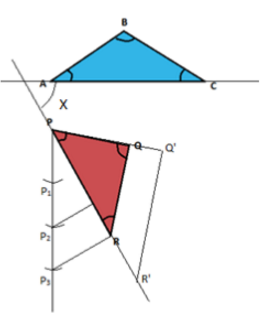1. If the perpendicular distance between AP is given, which vertices of a similar triangle would you find first?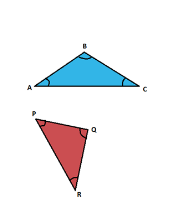1. R
2. Q
3. P
4. A

Solution: If the perpendicular distance of AP is given, then we would start the construction of a similar triangle by finding the position of the point P.

1. If you need to construct a triangle with point P as one of its vertices, which is the angle that you need to construct a side of the triangle?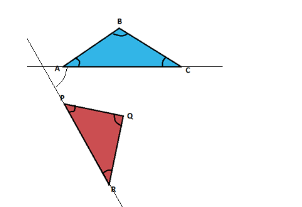1. ∠QPR
2. ∠RQP
3. ∠PRQ
4. Angle PR makes with AC

Answer: (D) Angle PR makes with AC.

Solution: Once we know the position of Point P, we need to find the orientation of the △PQR w.r.t △ABC. For that purpose, we need to know the angle of any one side that makes the original triangle. Therefore, among the given options, we will be able to determine the orientation of the △PQR if we know the angle at which side PR makes with side AC.

1. Match the following based on the construction of similar triangles, if the scale factor (m/n) is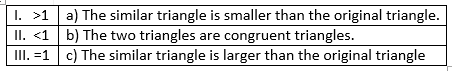1. I – c, II – a, III – b
2. I – b, II – a, III – c
3. I – a, II – c, III – b
4. I – a, II – b, III – c

Answer: (A) I – c, II – a III – b

Solution: The scale factor basically defines the ratio between the sides of the constructed triangle to that of the original triangle.

So, when we see the scale factor (m/n)>1, it means the sides of the constructed triangle are larger than the original triangle, i.e. the triangle constructed is larger than the original triangle.

Similarly, if scale factor (m/n) <1, then the sides of the constructed triangle are smaller than the original triangle, i.e. the constructed triangle is smaller than the original triangle.

When we have scale factor (m/n) = 1, then the sides of both the constructed triangle and the original triangle are equal.

When a pair of similar triangles have equal corresponding sides, then the pair of similar triangles can be called congruent because the triangles will have equal corresponding sides and equal corresponding angles.

1. The image of the construction of A,’ C’B, a similar triangle of ΔACB, is given below. Choose the correct option: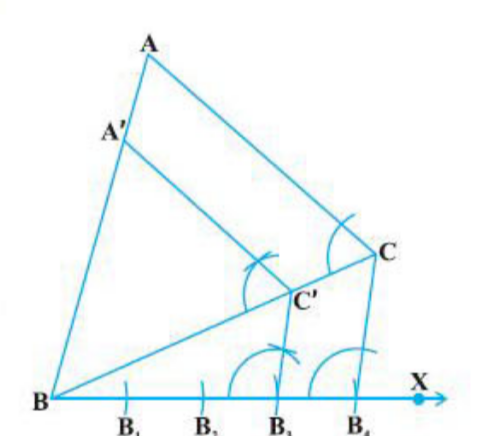1. ∠BA′C′ =∠BAC
2. ∠CAB=∠ACB
3. ∠A′BC′≠∠CBA
4. BA′/A′C′=BC/BC

Solution: As ΔABC∼ΔA′BC′

∠BA′C′=∠BAC (corresponding angles of similar triangles)

1. If a triangle similar to the given ΔABC with sides equal to 3/4 of the sides of ΔABC is to be constructed, then the number of points to be marked on ray BX is __.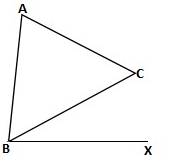1. 3
2. 4
3. 7
4. 6

Solution: In the ratio between sides 3/4, 4>3

⇒ The number of points to be marked on BX to construct similar triangles is 4.

1. The construction of similar polygons is similar to that of the construction of similar triangles. If you are asked to construct a parallelogram similar to a given parallelogram with a given scale factor, which of the given steps will help you construct a similar parallelogram?
1. Find a point on the larger side that divides it in the ratio of the given scale factor, and use the smaller side as the other parallel side to construct the parallelogram.
2. Find two points, one on the larger side and the other on the smaller side, using the given scale factor and using these scaled lengths to construct a similar parallelogram.
3. With one of the vertices as the centre and radius (scale factor multiplied by the length of the larger side), draw two arcs on the larger and smaller sides. Use these 2 points to construct the parallelogram.
4. None of these helps in constructing a similar parallelogram.

Answer: (B) Find two points, one on the larger side and the other on the smaller side, using the given scale factor and using these scaled lengths to construct a similar parallelogram.

Solution: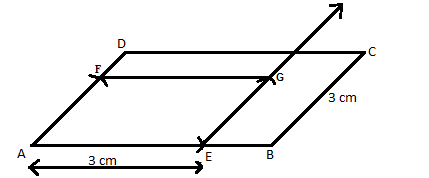The following steps will give you information on how to construct a parallelogram similar to ABCD.

Step 1: Find points E and F on longer and smaller sides, respectively, using the given scale factor.

Step 2: Draw a line from E parallel to the smaller side AD.

Step 3: Taking the length of AF and E as the centre, cut an arc on the line parallel to AD, and let this new point be G.

Step 4: Join EG and FG.

Step 5: AEFG is the required parallelogram.

Now, we have constructed the parallelogram AEFG ∼ ABCD.

### Construction of Tangents to a Circle

1. You are given a circle with radius ‘r’ and centre O. You are asked to draw a pair of tangents that are inclined at an angle of 60∘ with each other. Refer to the figure and select the option which would lead us to the required construction. d is the distance OE.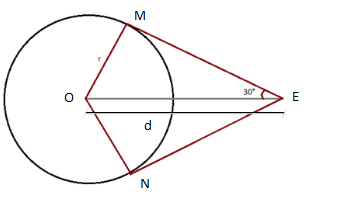1. Using trigonometry, arrive at d= √5 and mark E.
2. Using trigonometry, arrive at d= √3 and mark E.
3. Mark M and N on the circle such that ∠MOE = 60 ° and ∠NOE = 60 °
4. Construct the △MNO as it is equilateral

Answer: (C) Mark M and N on the circle, such that ∠MOE = 60 ° and ∠NOE = 60 °

Solution: The angle between the tangents is 60°, and OE bisects ∠MEN, ∠MEO = 30°.

Since △OME is right-angled at M, we realise that the ∠MOE = 60°. Since ∠MOE = 60°, we must have ∠NOE =60° and ∠MON = 120°. Hence, △MNO is NOT equilateral.

Next, since in △OME, sin30°= 1/2 = OM/OE = r/d, we have d = 2r.

Recalling that ∠MOE = 60°, the following are the steps of construction:

1. Draw a ray from the centre of O.

2. With O as centre, construct ∠MOE = 60° [constructing angle 60° is easy]

3. Now, extend OM, and from M, draw a line perpendicular to OM. This intersects the ray at E. This is the point from where the tangents should be drawn; EM is one tangent.

4. Similarly, EN is another tangent.

1. In the above scenario, after drawing the circle with radius R, what is the next thing to be constructed?
1. The point B
2. The point O
4. Tangent PO

Solution: Since we need to draw a circle with a radius ‘r’, we need the following points:

i) Centre of the smaller circle

ii) Radius of the smaller circle.

We have the radius ‘r’, but we need to adjust the centre on the line OA.

Realising that the tangents are common to both the circles, the radius of each circle at their point of contact being perpendicular to the common tangent, we can say that the radii are parallel. So, we also have the ratio PQ:QO because we have a pair of similar triangles.

But, before we can do all this, first, we need to have the line AO and, even before that, the point O. Only then we can draw tangents and then the inner circle. So, the next step would be determining the point ‘O’.

1. In reference to the above question, what would be the first thing to determine?
1. The radius of the circle ‘C.’
2. The radius of the circle ‘D.’
3. Centre of the circle ‘C.’
4. None of these

Answer: (C) Centre of the circle ‘C.’

Solution: Since we need to finally construct a circle of radius ‘R’ concentric to the previous circle, we need to determine the centre of these circles first, before proceeding with anything else.

The radius of the circle ‘D’ can be figured out after we get the radius of the first circle using the centre.

The radius of the circle ‘C’ can be found after finding the centre of this circle.

### Drawing Tangents to a Circle

1. Which of the following is not true for a point P on the circle?
1. Only 1 tangent can be drawn from point P
2. There are 2 tangents to the circle from point P
3. Perpendicular to the tangent passes through the centre
4. None of these

Answer: (B) There are 2 tangents to the circle from point P

Solution: Only one tangent can be drawn from a point on the circle, and the tangent is always perpendicular to the radius.

1. There is a circle with centre O. P is a point from there only one tangent can be drawn to this circle. What can we say about P?
1. O and P are co-incident points
2. P is on the circle
3. P is inside the circle
4. P is outside the circle

Answer: (B) P is on the circle.

Solution: Since only one tangent can be drawn, this point P should be present on the circle.

Any point on the circle is at a distance equal to the radius of the circle. So, OP is equal to the radius of the circle.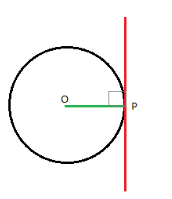1. A circle of radius r has a centre O. What is the first step to construct a tangent from a generic point P which is at a distance r from O?
1. With P as centre and radius > r, draw a circle and then join OP.
2. With P as centre and radius < r, draw a circle and then join OP.
3. With P as centre and radius = r, draw a circle and then join OP.
4. Join OP.

Solution: P is a point on the circle. We know that only one tangent can be drawn, and it is perpendicular to the line joining the centre of the circle O and the point of contact P.

So, the first step would be to join OP.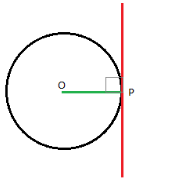### Dividing a Line Segment

1. A point C divides a line segment AB in the ratio 5:6. The ratio of lengths AB:BC is: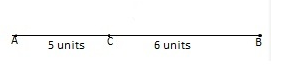1. 11:5
2. 11:6
3. 6:11
4. 5:11

Solution: Given AC/BC = 5/6

AB/BC= (AC+BC)/BC = (AC/BC) +1 = 5/6+1 = 11/6

So, the ratio is 11:6.

1. Point W divides the line XY in the ratio m:n. Then, the ratio of lengths of the line segments XY:WX, is
1. m+n:m
2. m+n:n
3. m:m+n
4. m:n

Solution: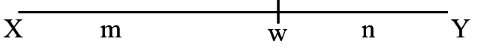XY/ XW= (XW/XW) + (WY/XW) = 1 + (n/m) = (m+n)/m = m+n: m

1. What is the ratio AC/BC for line segment AB following the construction method below? Step 1. A ray is extended from A, and 30 arcs of equal lengths are cut, cutting the ray at A1, A2,…A30Step 2. A line is drawn from A30 to B, and a line parallel to A30B is drawn, passing through point A17 and meeting AB at C.
1. 13:30
2. 13:17
3. 17:13
4. 17:30

Solution: Here, the total number of arcs is equal to m+n in the ratio m:n.

The triangles △ AA17C and △ AA30B are similar.

Hence, AC/AB = AA17/AA30 = 17/30

BC/AB = (AB−AC)/AB

BC/AB = 1 – 17/30 = 13/30

Hence, AC/BC = 17/13 = 17:13.

1. What is the ratio AC/BC for the following construction: A  line segment AB is drawn. A single ray is extended from A, and 12 arcs of equal lengths are cut, cutting the ray at A1, A2… A12. A line is drawn from A12 to B, and a line parallel to A12B is drawn, passing through point A6 and cutting AB at C.
1. 1:2
2. 1:1
3. 2:1
4. 3:1

Solution: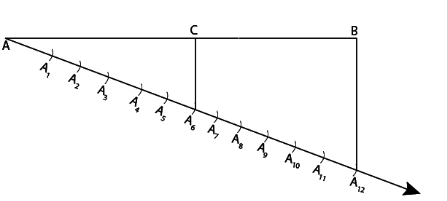In the construction process given, triangles △AA12B   △AA6C are similar.

Hence, we get AC/AB = 6/12 = 1/2.

By construction, BC/AB = 6/12 = 1/2.

AC/BC = (AC/AB)/ (BC/AB)

= (½)/ (½) = 1.

1. The basic principle used in dividing a line segment is:
1. Tangent to a circle
2. Congruency of triangles
3. Similarity of triangles
4. None of these

Solution: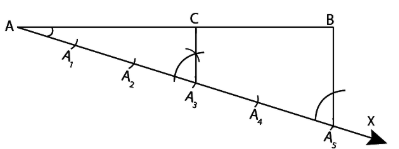The similarity of triangles is the basic principle used in dividing a line segment.

In this case, similar triangles ACA3 and ABA5 have been constructed to divide the line segment AB.

1. To divide a line segment, the ratio of division must be:
1. Negative and rational
2. Greater than 1
3. Less than 1
4. Positive and rational

Solution: The ratio of division must always be positive and rational. It can be greater than or less than 1.

Furthermore, Class 10 Maths Chapter 11 deals with various concepts of Construction, such as the Construction of Line Segments, Division of a Line Segment and Construction of a Circle, Construction of Tangents to a Circle using an analytical approach, and more.

Also, we have compiled below some extra questions for the students to solve:

### CBSE Class 10 Maths Chapter 11 Extra Questions

1. To divide a line segment PQ in the ratio 5:7, first, a ray PX is drawn so that ∠QPX is an acute angle, and then at equal distances, points are marked on the ray PX. If so, what will be the minimum number of these points?
(a) 5
(b) 7
(c) 12
(d) 10

2. If a line segment is divided in the ratio 2:3, calculate the number of parts it is divided into.
(a) 2/3
(b) 2
(c) 3
(d) 5

3.  If a pair of tangents have to be constructed from a point P to a circle of radius 3.5 cm. Then at what distance from the centre should the point be?
(a) 5 cm
(b) 2 cm
(c) 3 cm
(d) 3.5 cm

Stay tuned to BYJU’S for further updates on the CBSE Board exam. Also, download BYJU’S – The Learning App and subscribe to the YouTube channel to access interactive Maths and Science videos.